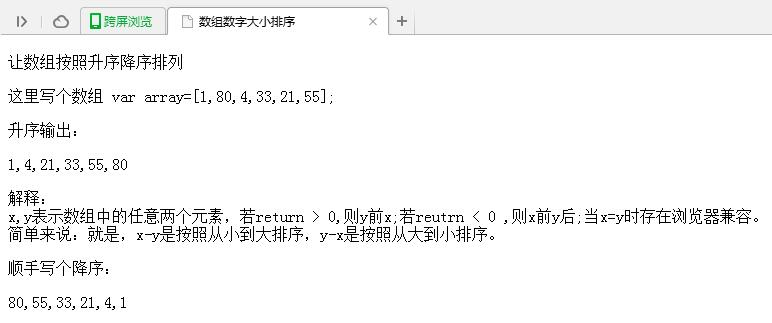• 升序排列代码 import java.util.Arrays; import java.util.Comparator; public class Test{ public static void main(String args[]) { //注意这里的数据类型必须为Integer而不能为int，因为只有Integer类继承了...
方法一：
升序排列代码
import java.util.Arrays;
import java.util.Comparator;
public class Test{
public static void main(String args[]) {
//注意这里的数据类型必须为Integer而不能为int，因为只有Integer类继承了Comparator接口而不是int
Integer[] _arr = new Integer[] { 5, 3, 1, 2, 4 };
Arrays.sort(_arr, new Comparator<Integer>() {
@Override
public int compare(Integer o1, Integer o2) {
return o1-o2;
}
});
for(int number: _arr)
System.out.print(number + " ");
System.out.println();
}
}

输出：1 2 3 4 5
降序排列代码
import java.util.Arrays;
import java.util.Comparator;
public class Test{
public static void main(String args[]) {
//注意这里的数据类型必须为Integer而不能为int，因为只有Integer类继承了Comparator接口而不是int
Integer[] _arr = new Integer[] { 5, 3, 1, 2, 4 };
Arrays.sort(_arr, new Comparator<Integer>() {
@Override
public int compare(Integer o1, Integer o2) {
return o2-o1;
}
});
for(int number: _arr)
System.out.print(number + " ");
System.out.println();
}
}

输出：5 4 3 2 1
方法二:
升序排列代码
public class Test{
public static void main(String args[]) {
int a[] = new int[]{1,5,6,4,8};
//作为中介元素
int temp = 0;
for (int i = 0; i < a.length; i++) {
for (int j = i+1; j < a.length; j++) {
if(a[i]>a[j]) {
temp = a[j];
a[j] = a[i];
a[i] = temp;
}
}
}
for(int number:a) {
System.out.print(number+" ");
}
}
}

降序排列代码
改变一下大小号就可以了
  public class Test{
public static void main(String args[]) {
int a[] = new int[]{1,5,6,4,8};
//作为中介元素
int temp = 0;
for (int i = 0; i < a.length; i++) {
for (int j = i+1; j < a.length; j++) {
if(a[i]<a[j]) {
temp = a[j];
a[j] = a[i];
a[i] = temp;
}
}
}
for(int number:a) {
System.out.print(number+" ");
}
}
}



展开全文排序 sort Array
• 主要介绍了JAVA基于Arrays.sort()实现数组升序降序,文中通过示例代码介绍的非常详细，对大家的学习或者工作具有一定的参考学习价值,需要的朋友可以参考下
• 分享给大家供大家参考，具体如下：数组数字大小排序让数组按照升序降序排列这里写个数组 var array=[1,80,4,33,21,55];升序输出：var array=[1,80,4,33,21,55];array.sort(function (x,y) {return x-y;});document....
本文实例讲述了JS实现数组按升序及降序排列的方法。分享给大家供大家参考，具体如下：数组数字大小排序让数组按照升序降序排列这里写个数组 var array=[1,80,4,33,21,55];升序输出：var array=[1,80,4,33,21,55];array.sort(function (x,y) {return x-y;});document.writeln(array);解释： x,y表示数组中的任意两个元素，若return > 0,则y前x;若reutrn < 0 ,则x前y后;当x=y时存在浏览器兼容。简单来说：就是，x-y是按照从小到大排序，y-x是按照从大到小排序。顺手写个降序：var array=[1,80,4,33,21,55];array.sort(function (x,y) {return y-x;});document.writeln(array);运行效果图如下：PS：这里再为大家推荐2款相关在线排序工具供大家参考使用：希望本文所述对大家JavaScript程序设计有所帮助。
展开全文• 我们在学习 Java 的过程中肯定会遇到对数组进行升序降序等排序问题，本节主要介绍如何实现 Java 数组升序降序Java 语言使用 Arrays 类提供的 sort() 方法来对数组进行排序。升序使用 java.util.Arrays 类中...
我们在学习 Java 的过程中肯定会遇到对数组进行升序或降序等排序问题，本节主要介绍如何实现 Java 数组的升序和降序。Java 语言使用 Arrays 类提供的 sort() 方法来对数组进行排序。升序使用 java.util.Arrays 类中的 sort() 方法对数组进行升序分为以下两步：导入 java.util.Arrays 包。使用 Arrays.sort(数组名) 语法对数组进行排序，排序规则是从小到大，即升序。假设在数组 scores 中存放了 5 名学生的成绩，现在要实现从低到高排列的功能。在这里使用 Arrays.sort() 方法来实现，具体代码如下：public static void main(String[] args) {// 定义含有5个元素的数组double[] scores = new double[] { 78, 45, 85, 97, 87 };System.out.println("排序前数组内容如下：");// 对scores数组进行循环遍历for (int i = 0; i < scores.length; i++) {System.out.print(scores[i] + "\t");}System.out.println("\n排序后的数组内容如下：");// 对数组进行排序Arrays.sort(scores);// 遍历排序后的数组for (int j = 0; j < scores.length; j++) {System.out.print(scores[j] + "\t");}}如上述代码所示，要对一个数组进行升序排列，只需要调用 Arrays.sort() 方法即可。运行后的输出结果如下所示。排序前数组内容如下：78.0    45.0    85.0    97.0    87.0排序后的数组内容如下：45.0    78.0    85.0    87.0    97.0降序在 Java 语言中使用 sort 实现降序有两种方法，简单了解即可。1)利用 Collections.reverseOrder() 方法(Collections 是一个包装类。大家可以学习《Java Collections类》一节详细了解)：public static void main(String[] args) {Integer[] a = { 9, 8, 7, 2, 3, 4, 1, 0, 6, 5 };    // 数组类型为IntegerArrays.sort(a, Collections.reverseOrder());for (int arr : a) {System.out.print(arr + " ");}}输出结果如下：9 8 7 6 5 4 3 2 1 02)实现 Comparator 接口的复写 compare() 方法，代码如下:public class Test {public static void main(String[] args) {/** 注意，要想改变默认的排列顺序，不能使用基本类型(int,double,char)而要使用它们对应的类*/Integer[] a = { 9, 8, 7, 2, 3, 4, 1, 0, 6, 5 };// 定义一个自定义类MyComparator的对象Comparator cmp = new MyComparator();Arrays.sort(a, cmp);for (int arr : a) {System.out.print(arr + " ");}}}// 实现Comparator接口class MyComparator implements Comparator {@Overridepublic int compare(Integer o1, Integer o2) {/** 如果o1小于o2，我们就返回正值，如果o1大于o2我们就返回负值， 这样颠倒一下，就可以实现降序排序了,反之即可自定义升序排序了*/return o2 - o1;}}输出结果如下所示。9 8 7 6 5 4 3 2 1 0注意：使用以上两种方法时，数组必须是包装类型，否则会编译不通过。在 Java 中实现数组排序的方式很多，除了利用以上的几种方法外，还可以编写自定义方法来实现自己的排序算法，有兴趣的读者可以尝试编写。
展开全文• 数组升序降序 package com.itcast.cn; import java.sql.Connection; import java.util.ArrayList; import java.util.Collections; import java.util.Comparator; public class demo1 { public static void ...
数组升序，降序
package com.itcast.cn;

import java.sql.Connection;
import java.util.ArrayList;
import java.util.Collections;
import java.util.Comparator;

public class demo1 {

public static void main(String[] args) {
ArrayList<Integer> list = new ArrayList<>();
System.out.println(list);

Collections.sort(list, new Comparator<Integer>() {
//覆盖重写

@Override
public int compare(Integer o1, Integer o2) {
// return o2-o1;//降序
return o1 - o2;//升序
}
});
System.out.println(list);

}
}



展开全文• import java.lang.*;import java.util.*;public class Main {public static void main(String args[]) {int a[] = new int[]{1, 4, 612, 333, -8, 2, -12, 4534, 0};for (int i = 0; i < a.length; i++) { //直接...
• java中对数组进行排序使用Array.sort() 这个默认是升序@Testpublic void index4(){int scores[] = new int[]{1,2,3,89,4};Arrays.sort(scores);for (int i:scores) {System.out.println(i);}}如果想降序怎么办呢？...
• 1 int[] x={1,6,4,8,6,9,12,32,76,34,23};...升序： 1 Arrays.sort(x); 降序： 1 resort(x); 2 public int[] resort(int[] num){ 3 Arrays.sort(num); 4 int[] resort=new int[num.length]; ...
• Java sort()数组排序(升序降序)我们在学习 Java 的过程中肯定会遇到对数组进行升序降序等排序问题，本节主要介绍如何实现 Java 数组升序降序Java 语言使用 Arrays 类提供的 sort() 方法来对数组进行排序。...
• 自然升序排序Java语言提供给我们Array.sort(int [] arr)对数组进行升序排列，代码如下：packagesong;importjava.util.Arrays;public classTestexample {public static voidmain(String[] args) {int[] a = {9, 8, 7,...
• // 功能：输入整型数组，对其元素按照升序降序进行排序  // 输入：pIntegerArray 需要排序的整型数组  // sortFlg 0:升序 1：降序  // 返回：没有重复数字的整型 Demo.java public static void ...华为OJ
• Integer[] a = {1, 6, 8, 5, 7, 13, 2}; Arrays.sort(a); for (int i = 0; i < a.length; i++) { System.out.print(a[i]+","); } System.out.println(); ...排序
• 每个节点应满足条件：若升序，则父节点大于或等于左右子节点* 的数据，若降序则反之。在这里选择升序，则根节点为最大值。堆排序需要反复两个步骤：构造堆结构和堆排序输出。*“筛”运算来调整节点数据，以满足堆...
• 默认升序package peng;import java.util.Arrays;public class Testexample {public static void main(String[] args) {int[] a = {9, 8, 7, 2, 3, 4, 1, 0, 6, 5};Arrays.sort(a);for(int arr:a) {System.out.print...
• //给定一个数组, 让数组升序 (降序) 排序. //每次尝试找到当前待排序区间中最小(或最大)的元素, 放到数组最前面(或最后面). public class Test9_bubbleSort { public static void main(String[] args){ int[] arr={9...
• 2.升序降序的不同只有两数比较时不同（max min） import java.util.*; public class main1 { public static void main(String[] args) { int[]A={0,-2}; int[]B={10,0,-1}; int len = A.length + B.length; ...
• Java升序容易，降序不易。基本类型不能降序，至少要是包装类。升序使用Arrays.sort()降序要么使用Collections.reverse，要么实现Comparator接口import java.util.Arrays;import java.util.Collections;import java....
• public class test9 { public static void main(String[] args)...原数组为："+"\n"+a+"\t"+a+"\t"+a+"\t"+a+"\t"+a+"\t"+a+&quo
• public class test8 { public static void main(String[] args){ int []a={...原数组为："+"\n"+a+"\t"+a+"\t"+a+"\t"+a+"\t"+a+"\t&qu
• 我们在学习 Java 的过程中肯定会遇到对数组进行升序降序等排序问题，本节主要介绍如何实现 Java 数组升序降序Java 语言使用 Arrays 类提供的 sort() 方法来对数组进行排序。升序使用 java.util.Arrays 类中...
• 先让数组升序排列 可以用 Arrays.sort(数组) 进行升序 算法 代码 import java.util.Arrays; public class testone { public static void main(String[] args) { int[] array = {1, 3, 4, 6, 7, 9}; // 数组...
• 输入样例： 3 20 18 15 10 75 72 45 输出样例： 94.50 C++可以使用结构体对平均价格进行降序排序 java需要使用对象针对平均价格进行降序排序 现在以java来解答： 首先建立月饼类 class 月饼 { Double num,sumprice...算法
• Java 用 sort 实现对数组升序降序排序一、升序二、降序 一、升序 使用 java.util.Arrays 类中的 sort() 方法对数组进行升序分为以下两步： 导入 java.util.Arrays 包。 使用 Arrays.sort(数组名) 语法对数组...数据结构 排序
• 我们在学习 Java 的过程中肯定会遇到对数组进行升序降序等排序问题，本节主要介绍如何实现 Java 数组升序降序Java 语言使用 Arrays 类提供的 sort() 方法来对数组进行排序。升序使用 java.util.Arrays 类中...
• 在调用Arrays.sort()对数组进行排序时，默认是升序排序的，如果想让数组降序排序，有下面两种方法： 利用Collections的reverseOrder import java.util.*; public class Main { public static void main(String[]......

# java数组升序降序java 订阅